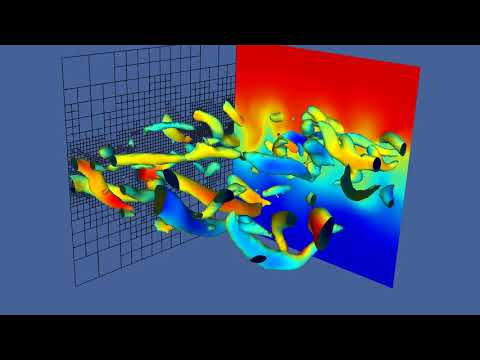# sandbox/Antoonvh/kh3d.c

## A Kelvin-Helmholtz instability in 3D

On this page the set-up to simulate a simple shear instability in the 3D is shown. The set-up is somewhat similar to the 2D set-up. However, due to the increased degrees of freedom, the results are dramatically different. Furthermore, the addition of the extra dimension causes the simulation to be much more expensive. Therefore it is run off-line on my laptop (taking \approx 3 hours in MPI mode). We use an adaptive grid, and visualize the results with the famous \lambda_2 vortex-detection algorithm.

#include "grid/octree.h"
#include "navier-stokes/centered.h"
#include "lambda2.h"

The Reynoldsnumber based on the periodic lengthscale (\mathcal{L}) as introduced on the 2D page, is reduced to be Re_{\mathcal{L}}=5000, to keep the simulation feasable. In order to promote the growth of the instability in this more viscous regime we add a few perturbation modes to the fluid layers’ interface. The maximum resolution corresponds to a 256^3 grid.

#define border (y+0.01*sin(18*M_PI*x)+0.005*cos(10*M_PI*x)+0.01*sin(12*M_PI*z)+0.005*cos(14*M_PI*z)+0.01*exp(-((x*x)+(y*y)))>0)

int maxlevel = 8;
double f = 0.001;
double ue=0.04;
const face vector muc[]={0.0002,0.0002};

Periodicity of the solution in the span wise and stream wise direction are set before the simulation in started.

int main(){
periodic(left);
periodic(front);
X0=Z0=Y0=-L0/2;
N=16;
run();
}

The flow is initialized in two layers with a normalized velocity difference. Furtheremore, the earlier defined “border” is used to prime it for the growth of the instability.

event init(i=0){
DT=0.0041;
mu=muc;
refine(sq(y)<0.01 && level < (maxlevel-1));
refine(sq(y)<0.005 && level < (maxlevel));
foreach(){
u.x[]=-0.5+border;
foreach_dimension()
u.x[]+=0.01*noise();
}
boundary(all);
}

256^3 grid points is a bit much to handle for my laptop. Fortunately, we can now combine periodic boundaries with with anisotropic, adaptive meshing.

event adapt(i++)
adapt_wavelet((scalar *){u},(double[]){ue,ue,ue},maxlevel);

## Output and results

We limit ourselves to a visualization of the vortex detection algorithm based on the \lambda_2 iso surface.

event gfsview(t+=0.02;t<=10){
scalar l[];
lambda2(u,l);
static FILE * fpm = popen("gfsview-batch3D ekman.gfv | ppm2mp4 > KH8.mp4","w");
output_gfs(fpm);
fprintf(fpm, "Save stdout {format = PPM width = 1920 height = 1080 }\n");The movie shows the evolution of a slice of the used numerical mesh. For a more qualitative analysis, the ratio of the number of grid cells in an equidistant grid with 256^3 point and the used number of grid cells (i.e \Pi>1) is calculated and plotted below…

  static FILE * fpo = fopen("reduc","w");
int n=0;
foreach(reduction(+:n))
n++;
fprintf(fpo,"%d\t%g\t%d\t%g\n",i,t,n,(double)((1<<(maxlevel*3))/n));
fprintf(ferr,"%d\t%g\t%d\t%g\n",i,t,n,(double)((1<<(maxlevel*3))/n));
set xlabel 'iteration'
set ylabel 'Pi'
set size square
set key off
plot 'kh3d.reduc' u 1:4 w l lw 3(script)

}